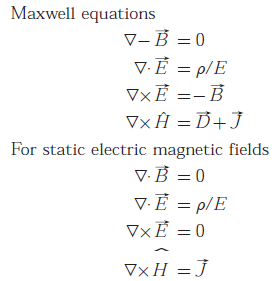# EC GATE 2008 - Online Test

Q1. Which of the following is NOT associated with a p - n junction ?
Answer : Option D
Explaination / Solution:

Channel length modulation is not associated with a p - n junction. It is being associated with MOSFET in which effective channel length decreases, producing the phenomenon called channel length modulation.

Q2. Which of the following is true?
Answer : Option A
Explaination / Solution:

Trivalent impurities are used for making p - type semiconductors. So, Silicon wafer heavily doped with boron is a p+ substrate.

Q3. In the following limiter circuit, an input voltage Vi = 10 sin100πt is applied. Assume that the diode drop is 0.7 V when it is forward biased. When it is forward biased. The zener breakdown voltage is 6.8 V
The maximum and minimum values of the output voltage respectively are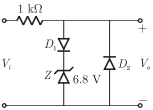Answer : Option C
Explaination / Solution:
No Explaination.

Q4. A silicon wafer has 100 nm of oxide on it and is furnace at a temperature above 10000 C for further oxidation in dry oxygen. The oxidation rate
Answer : Option D
Explaination / Solution:
No Explaination.

Q5. The drain current of MOSFET in saturation is given by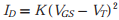where K is a constant.
The magnitude of the transconductance gm is
Answer : Option B
Explaination / Solution:Q6.
For the circuit shown in the following figure, transistor M1 and M2 are identical NMOS transistors. Assume the M2 is in saturation and the output is unloaded.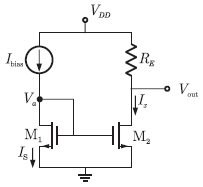The current Ix is related to Ibias as

Answer : Option B
Explaination / Solution:Q7.
The measured trans conductance gm of an NMOS transistor operating in the linear region is plotted against the gate voltage VG at a constant drain voltage VD. Which of the following figures represents the expected dependence of gm on VG ?
Answer : Option C
Explaination / Solution:
No Explaination.

Q8. For a Hertz dipole antenna, the half power beam width (HPBW) in the E-plane is
Answer : Option C
Explaination / Solution:

The beam-width of Hertizian dipole is 1800 and its half power beam-width is 900

Q9. Consider the following circuit using an ideal OPAMP. The I-V characteristic of the diode is described by the relationwhere VT = 25 mV, I0 = 1μ A and V is the voltage across the diode (taken as positive for forward bias). For an input voltage Vi = -1 V, the output voltage V0 is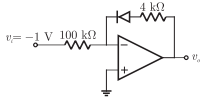Answer : Option B
Explaination / Solution:Q10. For static electric and magnetic fields in an inhomogeneous source-free medium, which of the following represents the correct form of Maxwell’s equations ?
Answer : Option D
Explaination / Solution: